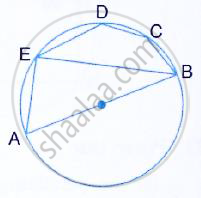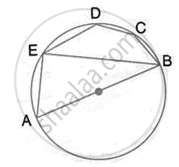Share

In the Given Figure, Ab is a Diameter of the Circle. Chord Ed is Parallel to Ab and ∠Eab = 63°. Calculate (I) ∠Eba (Ii) ∠Bcd - ICSE Class 10 - Mathematics

ConceptArc and Chord Properties - Angle in a Semi-circle is a Right Angle

Question

In the given figure, AB is a diameter of the circle. Chord ED is parallel to AB and ∠EAB = 63°. Calculate
(i) ∠EBA (ii) ∠BCDSolution(i) ∠AEB = 90
(Angle in a semicircle is a right angle)
Therefore ∠EBA = 90° - ∠EAB = 90° - 63° = 27°

(ii) AB || ED
Therefore ∠DEB = EBA = 27° (Alternate angles)

Therefore BCDE is a cyclic quadrilateral
Therefore ∠DEB + ∠BCD = 180°
[pair of opposite angles in a cyclic quadrilateral are supplementary]

Therefore ∠BCD =180° - 27°   =153°

Is there an error in this question or solution?

Video TutorialsVIEW ALL 

Solution In the Given Figure, Ab is a Diameter of the Circle. Chord Ed is Parallel to Ab and ∠Eab = 63°. Calculate (I) ∠Eba (Ii) ∠Bcd Concept: Arc and Chord Properties - Angle in a Semi-circle is a Right Angle.
S